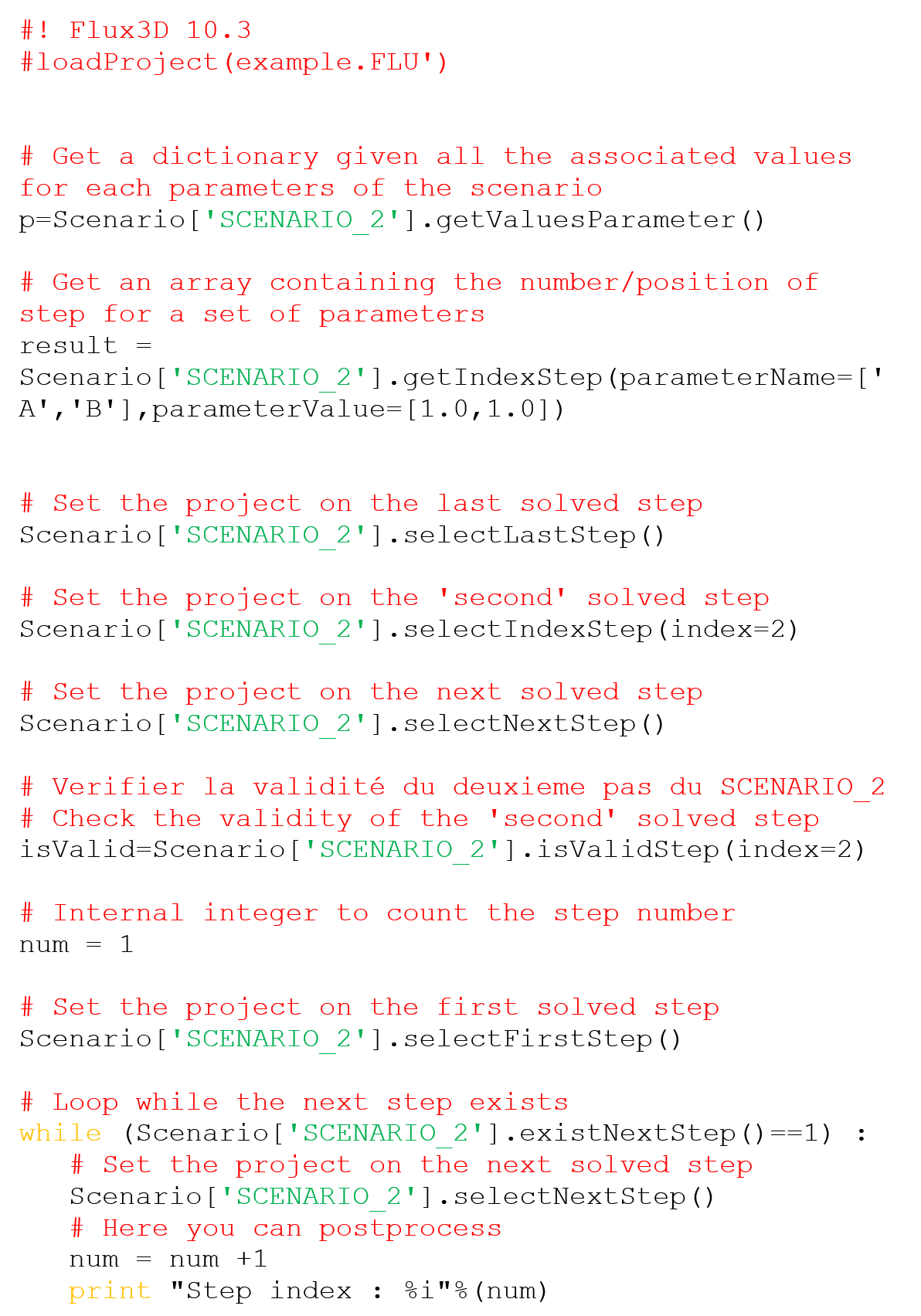# How to Get the Steps of a Scenario?

## Introduction

Some API have been implemented to allow the user to select steps to quickly postprocess. All methods have been added on the structure SCENARIO.

## API list

• isPostprocessingOK()
• getValuesParameter()
• getIndexStep (String[] parameterNames, double[] values)
• selectIndexStep(int index)
• selectFirstStep()
• selectLastStep()
• selectNextStep()
• existNextStep()
• isValidStep(int index)

## isPostprocessingOK

• Initial state: the scenario exists
• Input: none
• Output:
• valid: an integer (0 or 1) for (is not OK or OK)
• Final state: no change

## getValuesParameter

• Initial state: the scenario is solved and is multi step
• Input: none
• Output:
• a dictionary {key=<String>parameterName, values = <double>parameterValues}
• Final state: return all parameters values except if scenario has no parameter

## getIndexStep

• Initial state: the scenario is solved and is multi step
• Input (use the dictionary obtained by getValuesParameter)
• parameterName: String array dim N (N <= parameter number in the scenario)
• parameterValue: a double array dim N (N < = parameter number in the scenario)
• Output:
• an integer array corresponding to step index
• Final state: no change

## selectIndexStep

• Initial state: the scenario is solved and is multi step
• Input (use the integer array gets by getIndexStep)
• index: the index of step
• Output: none
• Final state: the project is changed on the required step

## selectFirstStep

• Initial state: the scenario is solved and is multi step
• Input: none
• Output: none
• Final state: the project is changed on the first step scenario

## selectLastStep

• Initial state: the scenario is solved and is multi step
• Input: none
• Output: none
• Final state: the project is changed on the last step scenario

## selectNextStep

• Initial state: the scenario is solved
• Input: none
• Output: none
• Final state: the project is changed on a step of the scenario

## existNextStep

• Initial state: the scenario is solved
• Input: none
• Output:
• exist: an integer (0 or 1) for (no exist or exist)
• Final state: no change

## isValidStep

• Initial state: the scenario is solved
• Input (use the integer array gets by getIndexStep)
• index: the index of step
• Output:
• state: an integer (0 or 1) for (invalid or valid)
• Final state: no change

## Example